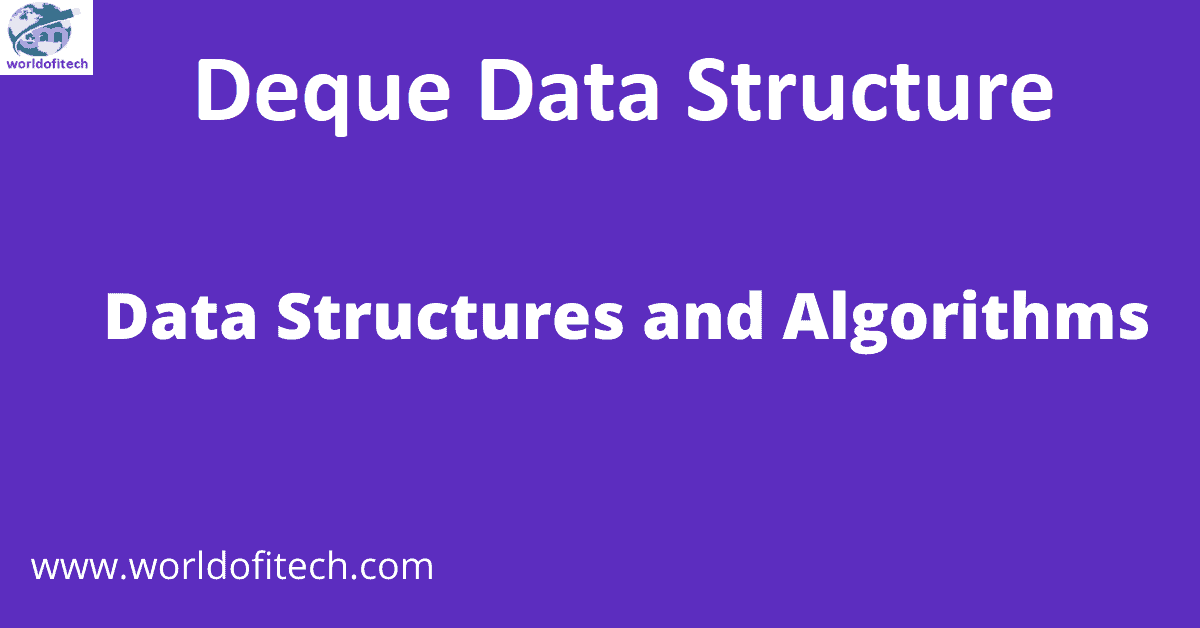Contents

#### Deque Data Structure

In this tutorial, you will learn what a double-ended queue (deque) is. Likewise, you will discover working instances of various ...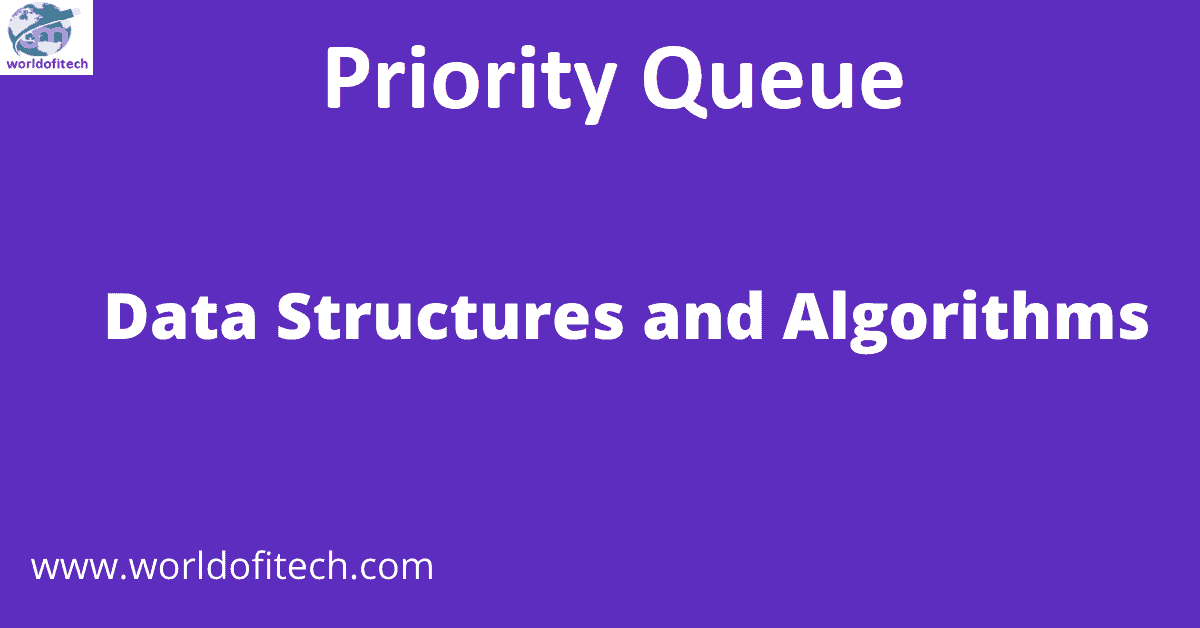#### Priority Queue

In this tutorial, you will learn what a priority queue is. Likewise, you will learn about its implementations in Python, ...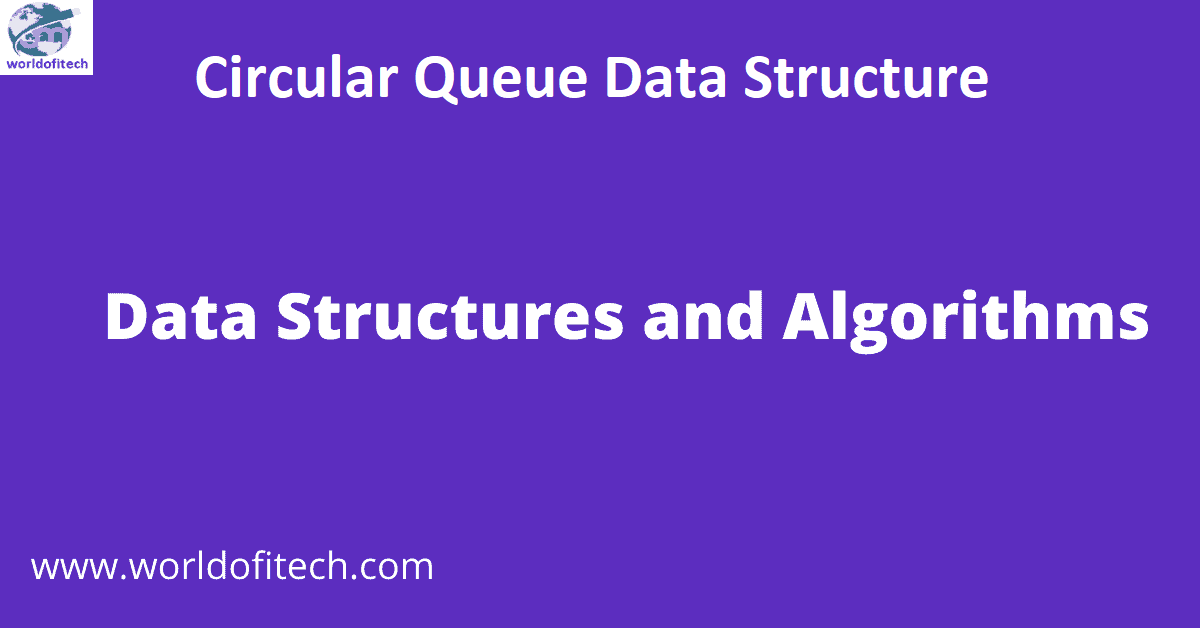#### Circular Queue Data Structure

In this tutorial, you will learn what a circular queue is. Additionally, you will discover the implementation of the circular ...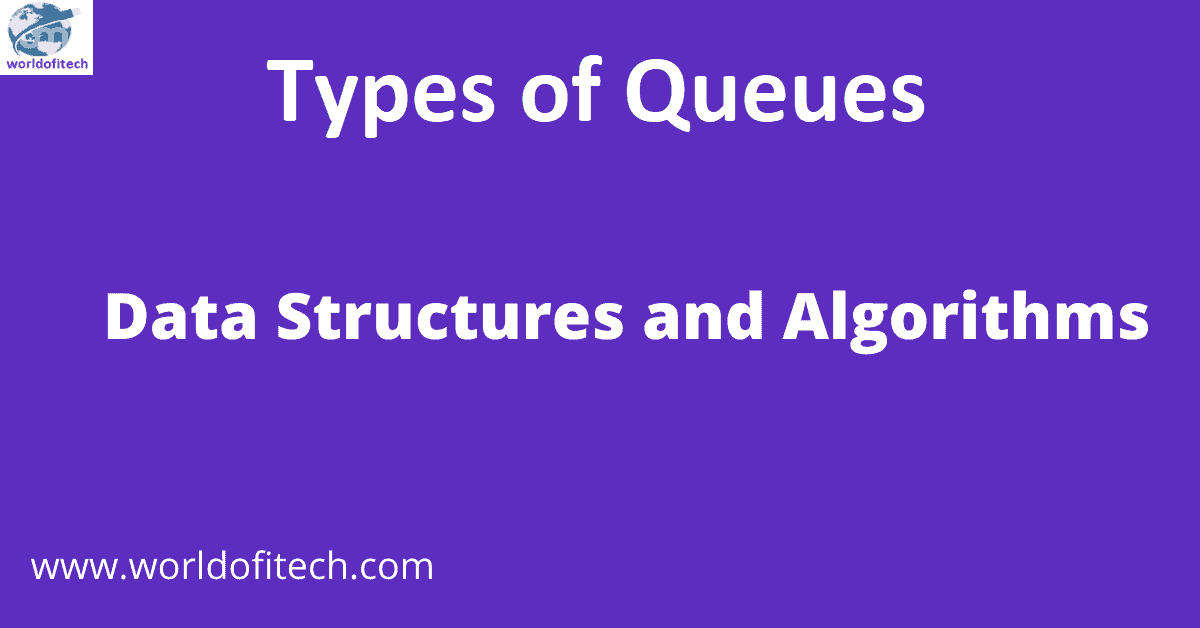#### Types of Queues

In this tutorial, you will learn various types of queues along with illustrations. What is a queue? A Queue is ...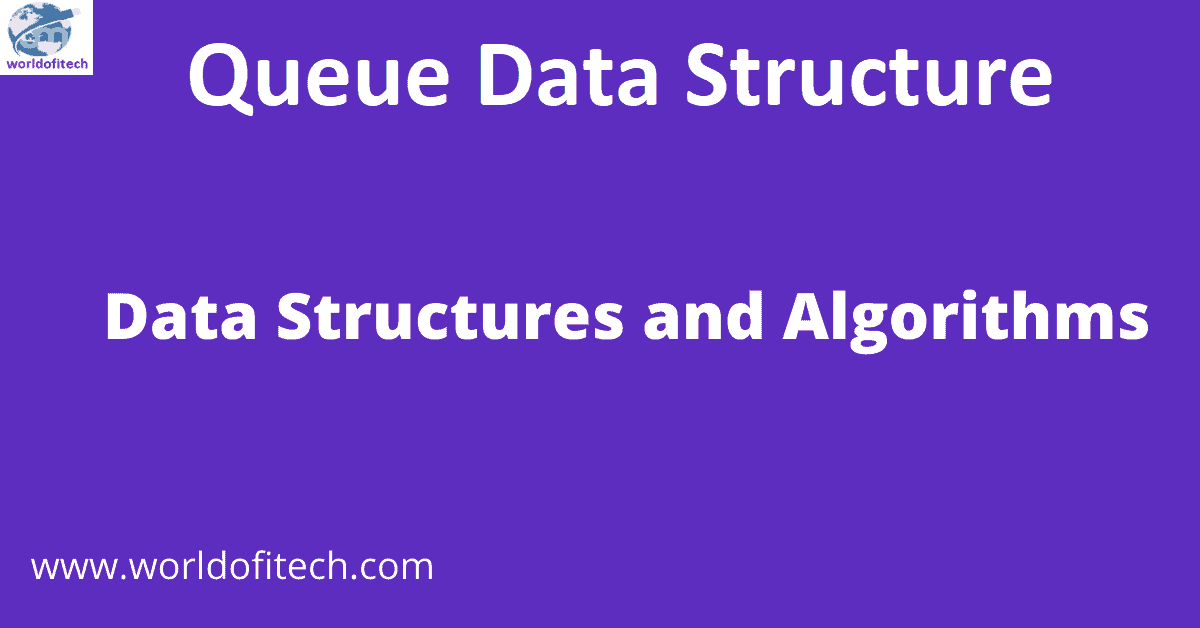#### Queue Data Structure

In this tutorial, you will learn what a queue is. Additionally, you will discover the implementation of the queue in ...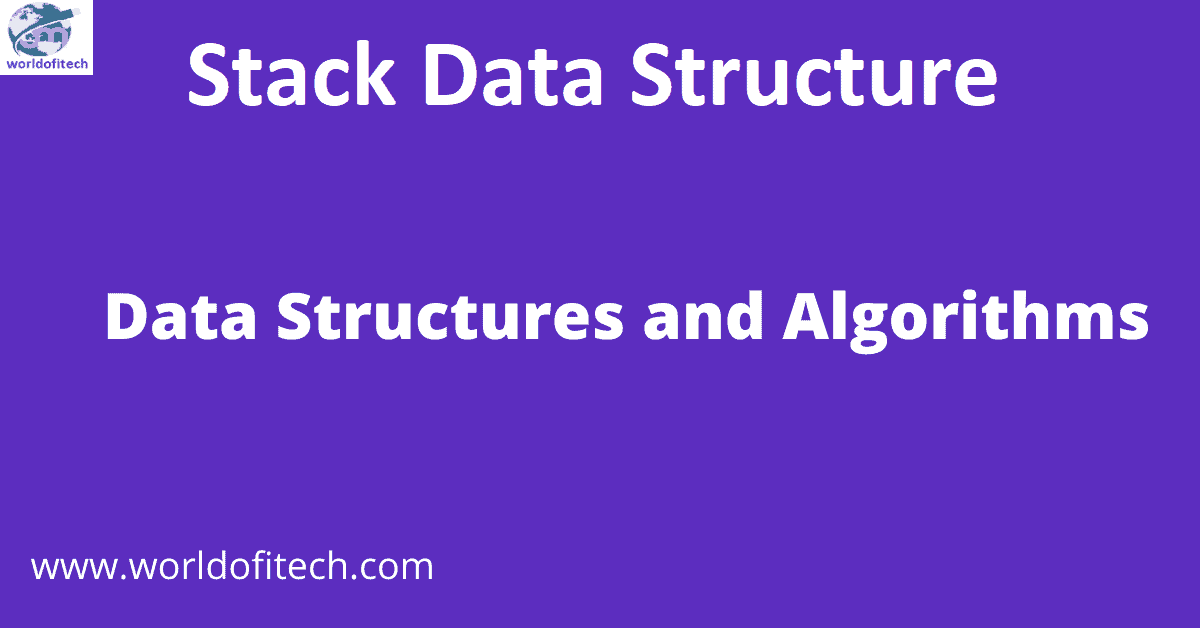#### Stack Data Structure

In this tutorial, you learn about the stack data structure and its implementation in Python, Java, and C/C++. What is ...